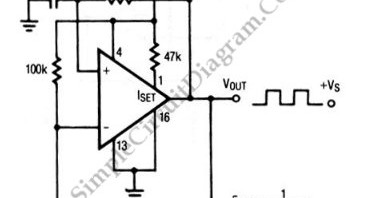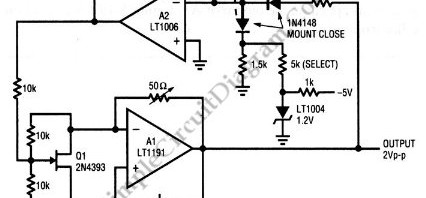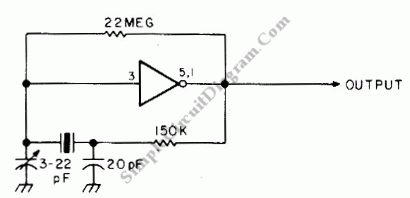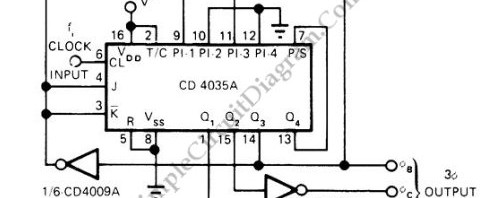## Square Wave Oscillator using Comparator/Op-AmpThe circuit shown in this schematic diagram is a square-wave oscillator circuit.  The main component of this oscillator circuit is LP165/365 comparator. As timing component, it uses capacitor and resistor to determine the frequency operation, generating square waves signal. Here is the schematic diagram of the circuit: We can change the values of R and C to produce different frequencies. […]

## Electronic Gain Control in Quartz-Stabilized OscillatorThis is a quartz-stabilized oscillator circuit with electronic gain control. Replacing the common filament lamp  for amplitude stabilization, this circuit use an electronic gain control inside the stabilization loop. A 50-Ω variable resistor should be trimmed until the output level show 2-Vpp magnitude oscillations op-amp A1 output. Here is  the schematic diagram of the circuit: [Circuit’s schematic diagram source: Linear […]

## Crystal Oscillator with CMOS InverterThis is an astable multivibrator  (oscillator) circuit using CMOS inverter. This circuit uses CD4007 or MC14007. This circuit has operating frequency range of 10kHz to 10MHz.  The frequency is not determined only by the crystal, but fine tuned further by C and R that determines the exact operating frequency of this circuit. For proper operation, Pin 1 and 5 should […]